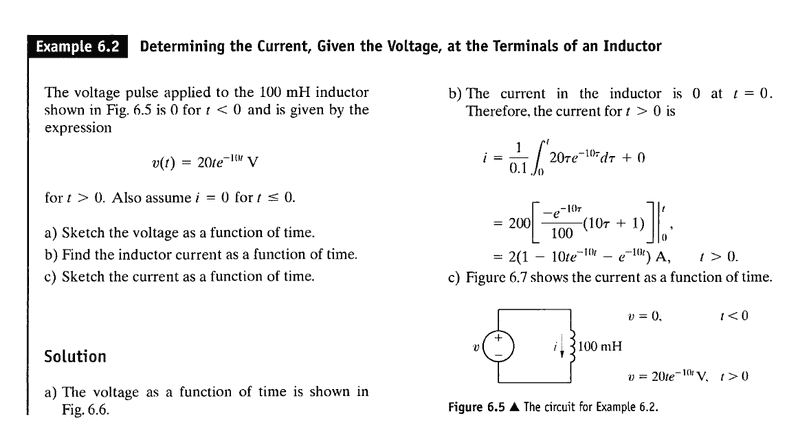# Integration Problem

jayanthd
I know ∫(xe$^{ax}$) dx = x (e$^{ax}$ / a) - (1/a) ∫e$^{ax}$ . 1 dx

= x (e$^{ax}$ / a) - (1/a) (e$^{ax}$ / a)

= (e$^{ax}$ / a) (x - 1/a)

i.e, integral of two functions = (first function) (integral of second function) - ∫(integral of second function) (differential of first function)

This is not a homework. I am a working professional and I need help in solving a problem.

The solution I need is for

(1/0.1) ∫20te$^{-10t}$ dt between limits 0 and 20 us. limits can be taken as 0 to t. I don't need numerical solution.

t = $\tau$

dt = d$\tau$

integral becomes

200 ∫$\tau$e$^{-10\tau}$ d$\tau$ between limits 0 and t

it becomes 200 [ $\tau$ (e$^{-10\tau}$ / - 10) + (1/10) ∫e$^{-10\tau}$ . 1 d$\tau$

= 200 [ $\tau$ (e$^{-10\tau}$ / - 10) + (1/10) (e$^{-10\tau}$ / - 10)]

= 200 [ $\tau$ (e$^{-10\tau}$ / - 10) - (1/100) e$^{-10\tau}$]

I know I have to apply limits to the two e$^{-10\tau}$

I want to know should I apply limits also to $\tau$ which is at the beginning of the solution (here... = 200 [ $\tau$ ...) ?

Last edited:

## Answers and Replies

Homework Helper
So you have the indefinite integral
$$\int \tau e^{-10 \tau} \, d\tau = - \tau e^{-10\tau} / 10 - e^{-10\tau} / 100 = -\frac{e^{-10\tau}}{10} \left( \tau - \frac{1}{10} \right)$$
which looks correct to me (I've rewritten it slightly to look a bit better).

Now evaluate that expression at ##\tau = t##, and at ##\tau = 0##, and subtract the result.

Staff Emeritus
Homework Helper
Yes, everywhere there is a τ, you must substitute the limits.

jayanthd
Thank you CompuChip and SteamKing.

CompuChip my question was

I know I have to apply limits to the two (e−10τ / - 10)

I want to know should I apply limits also to τ which is at the beginning of the solution (here... = 200 [ τ ...) ?

Yes, CompuChip the solution you gave is what I have. I was updating my first post to show the solution in the form you gave.

SteamKing gave the answer.

Thank you both of you.

So the limits are applied to whole solution and not just the integrals. Right? i.e., Even though $\tau$ is not integrated or differentiated in the process.

Edit: CompuChip I think you made a mistake.

Is it not

-e$^{-10\tau}$ / 10 ($\tau$ + 1/10)

I get ($\tau$ + 1/10) in the brackets

yours is ($\tau$ - 1/10)

You made a mistake in the sign?

I was referring the book "Electric Circuits 9th edition by Nilsson and Riedel" page no 178.#### Attachments

Last edited:
Homework Helper
Edit: CompuChip I think you made a mistake.

Is it not

-e$^{-10\tau}$ / 10 ($\tau$ + 1/10)

I get ($\tau$ + 1/10) in the brackets

yours is ($\tau$ - 1/10)

You made a mistake in the sign?

Yes, you are right. Good catch!

I mean, of course I was just checking if you were paying attention.